# Switching Regulators, Feedback Control Circuits

#### twinsen

Joined Jul 25, 2015
5
Hello.

I have to say right away that this is probably a very stupid question, but I really need to ask. It's about switching power supplies in general, feedback contorl in parcitular. I've been using them quite successfully for quite a while, but never got to thinking about it. The question is this.

The purpose of the error amplifiers in the feedback control circuitry is te eventually bring the output voltage (divided according to the requirements) as close as possible to the reference voltage. If that is so, that means that whenever the difference between these voltages is zero (which is the point of it all), the resulting duty cycle of the pwm controller seems to always be the same. In that case, how does it come about that by varying the resistor divider ratio one gets to acquire different output voltages?

#### AnalogKid

Joined Aug 1, 2013
10,305
The purpose of the error amplifiers in the feedback control circuitry is te eventually bring the output voltage (divided according to the requirements) as close as possible to the reference voltage. If that is so, that means that whenever the difference between these voltages is zero (which is the point of it all), the resulting duty cycle of the pwm controller seems to always be the same.
Nope. The difference between the two voltages is zero *at the opamp input pins.* But the opamp output is not zero, it is at some voltage that is proportional to the desired output voltage. The opamp's feedback loop includes the voltage amplifier, PWM, switching transistors, transformer, rectifier, output voltage divider, and communication back across the isolation barrier. So for a topology that has a constant reference voltage and variable output divider, everything changes so that the opamp pins come to a zero voltage difference.

ak

•Hypatia's Protege

#### Lestraveled

Joined May 19, 2014
1,946
1 - The pulse width must change to produce a different voltage. It has to. Perhaps you are not measuring it with enough resolution.

2 - There two ways to change the output voltage, change the reference voltage or, change the scaling of the feedback. In both cases the input to the op-amp is the same, the - input equals the + input. It will just take a different output voltage to get to that "balance" point.

#### twinsen

Joined Jul 25, 2015
5
Hello again.

Thank you very much for all your replies. All I can say is, at this point, I get it in principle. But still not quite there. Let's forget about how the real error amplifiers are buit. Let's instead assume that everything is ideal, and so is the error amplifier - it is an ideal differential amplifier with a fixed gain: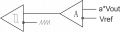This is a very crude image of what is going on. The "a" is the ratio of the resistor divider. Our goal is to choose "a" so that the resulting voltage fed back to the EA be equal to Vref. And if "a*Vout = Vref", then "Vout = Vref/a". But in that case the output of the EA is always the same, and so is the duty cycle, and so is the Vout.

I know it all looks stupefyingly stupid to you. But I just can't get there, it's really hilarious, but it's bugging me like hell. Could you please, if it's not too much to ask, explain all this to me using some real working equations?

Thank you very much in advance.

#### Jony130

Joined Feb 17, 2009
5,445
I can't say much about your digram because we do not have a feedback loop in your diagram. Also error amplifier in switching regulator work exactly the same as in the linear regulator.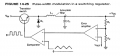The triangle wave is fed into the non inverting input of a second comparator. An error amplifier is used to monitor the filtered dc output of the low-pass filter. The output of the error amplifier is directed into the inverting input of the comparator. The comparator develops pulses at its output which are used to drive the transistor switch.
Let us focus our attention on the operation of the comparator and the error amplifier. The output of the error amplifier is directly proportional
to the dc voltage appearing at the output. The output of the error amplifier serves as the reference voltage for the comparator. If the reference
voltage is equal to one-half of the triangle's peak voltage. The output of the comparator will be a square wave [which is a rectangular wave with a 50% duty cycle.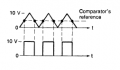If the output voltage increases, the output of the error amplifier will also increase. This raises the comparator's reference and lowers the duty cycle of its output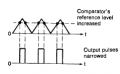If the output voltage is reduced, the output of the error amplifier will also decrease. This lowers the comparator's voltage reference and raises the duty
cycle of its output.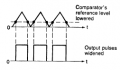In both cases the duty cycle is adjusted to counter the changes in the output voltage in order to maintain a constant output voltage.

http://www.planetanalog.com/author.asp?section_id=483&doc_id=559556

•absf

#### OBW0549

Joined Mar 2, 2015
3,566
The "a" is the ratio of the resistor divider. Our goal is to choose "a" so that the resulting voltage fed back to the EA be equal to Vref. And if "a*Vout = Vref", then "Vout = Vref/a". But in that case the output of the EA is always the same, and so is the duty cycle, and so is the Vout. I know it all looks stupefyingly stupid to you. But I just can't get there, it's really hilarious, but it's bugging me like hell.
No, it's not stupefyingly stupid; it's just that you've got yourself caught in a logical trap and haven't spotted it yet.

And that trap is the invalid assumption that the differential input to your error amp is zero. It is NOT zero, because the gain of the error amplifier is finite. The gain is large, but it is not infinite; and the differential error amp input is small, but not zero.

If we take "a" as the resistor divider ratio, as you have it, and "A" as the gain of the error amplifier together with everything that follows it (PW modulator, transistor power switch and catch diode, LC filter, etc.), then doing a little algebra on the input expressions gives us

Vout = Vref * (1 / ((1 / A) + a))​

which, if we raise A toward infinity, approaches

Vout = Vref * (1/a)​

in the limit, as we would expect.

With a little more algebra, you can derive an expression for the actual differential voltage on the input of the error amplifier; I'll leave that up to you.

Last edited:
•absf

#### twinsen

Joined Jul 25, 2015
5
Jony130.

Thank you for the top-notch diagrams that you cared enough to provide and I failed to. They are very helpful to further my line of questioning and point out the source of my puzzlement.

Let's say for the sake of being specific, that Vin = 5V, Vref = 1.25V. Let's also assume that all the components that realize switches (the bipolar and the diode) are pretty much ideal. Also, let's assume first that we need to see Vdc = 2.5V at the output. For that to happen, the resistors should be chosen so as to Vdc*R2/(R1 + R2) = Vref = 1.25V. At least this is the common design imperative. After we have chosen the resistors, we are supposed to see 50% duty cycle rectangular wave at the transistor's gate (base in this case). That gives us just what we need - Vdc = 0.5*Vin = 2.5V.

Now, let's see if we can get another DC level at the output, like Vdc = 1V. As before, Vdc*R2/(R1 + R2) = Vref. Resistance values will be different this time, but that should not change the fact that that we're aming for here is that the error be equal to zero. That, again, gives au the 50% duty cycle... and there is obviously a problem. Actually, of course, the duty cycle won't be 50%, because balancing equations won't allow that. But it sure won't be 20%, as it is supposed to.

Was I successful in describing my ridiculous problem? I am really ashamed to have it in the first place. Please help me get out of this.

#### twinsen

Joined Jul 25, 2015
5
OBW0549.

Of course you are absolutely right. I have no idea how I got myself into this mess. This stupid glitch got me really worried about myself. I guess I'd better get myself started on psych meds.

Thanks to all of you who were patient enough to see me through this. My appreciation for this community is beyond words.

#### OBW0549

Joined Mar 2, 2015
3,566
Don't feel bad; I've got myself caught in that same trap a few times, and eventually learned to watch out for those simplifying assumptions we often make that give results that are close, but not exact.

#### Jony130

Joined Feb 17, 2009
5,445
Now, let's see if we can get another DC level at the output, like Vdc = 1V. As before, Vdc*R2/(R1 + R2) = Vref. Resistance values will be different this time, but that should not change the fact that that we're aming for here is that the error be equal to zero. That, again, gives au the 50% duty cycle... and there is obviously a problem. Actually, of course, the duty cycle won't be 50%, because balancing equations won't allow that. But it sure won't be 20%, as it is supposed to..
Error amplifier just like an ordinary Op Amp will do whatever it can do make (changing the output voltage) to bring Vref = Vdc * R2/(R1 + R1).
So for Vref = 1.25V and Vin = 5V we will get Vdc = 2.5V only if duty cycle of a buck converter is equal to 50% (only in CCM this is true).
But now If we change the resistor value to get 2V at the output (R1 = 12K R2 = 20K ) (It is impassible to get 1V at the output because Vdc cannot be lower than Vref). The EA will change his output voltage and this will change the duty cycle to the new value 40%. Because only if D = 40% , Vdc can be equal to 2V and Vdc*R2/(R1 + R2) = Vref = 1.25V. For example if Vdc is 2.1V the voltage at divider output is 1.31V. So EA will still be changing his output voltage and this will change the duty cycle also. And the EA will stop changing his output voltage and the duty cycle only if Vref = Vdc * R2/(R1 + R1).
http://forum.allaboutcircuits.com/t...ns-but-im-troubled-by-them.64696/#post-444315 (start from "The more accurate explanation")

•absf

#### crutschow

Joined Mar 14, 2008
32,054
As someone famous once said
Don't feel bad; I've got myself caught in that same trap a few times, and eventually learned to watch out for those simplifying assumptions we often make that give results that are close, but not exact.
Certainly it's a real art to determine how much you can simplify a problem without adversely affecting the results.
That would seem to be an important thing that all engineers must learn.

#### MrAl

Joined Jun 17, 2014
10,079
Jony130.

Thank you for the top-notch diagrams that you cared enough to provide and I failed to. They are very helpful to further my line of questioning and point out the source of my puzzlement.

Let's say for the sake of being specific, that Vin = 5V, Vref = 1.25V. Let's also assume that all the components that realize switches (the bipolar and the diode) are pretty much ideal. Also, let's assume first that we need to see Vdc = 2.5V at the output. For that to happen, the resistors should be chosen so as to Vdc*R2/(R1 + R2) = Vref = 1.25V. At least this is the common design imperative. After we have chosen the resistors, we are supposed to see 50% duty cycle rectangular wave at the transistor's gate (base in this case). That gives us just what we need - Vdc = 0.5*Vin = 2.5V.

Now, let's see if we can get another DC level at the output, like Vdc = 1V. As before, Vdc*R2/(R1 + R2) = Vref. Resistance values will be different this time, but that should not change the fact that that we're aming for here is that the error be equal to zero. That, again, gives au the 50% duty cycle... and there is obviously a problem. Actually, of course, the duty cycle won't be 50%, because balancing equations won't allow that. But it sure won't be 20%, as it is supposed to.

Was I successful in describing my ridiculous problem? I am really ashamed to have it in the first place. Please help me get out of this.

Hi,

Sometimes the accepted nomenclature for different electrical objects interferes with the correct understanding of the function of that object. Here we have a couple of problems that if looked at in a more correct light will lead to a quicker understanding.

First, the Error Amplifier:
This isnt really just an amplifier, it's an integrator with amplification. Integrators differ from pure linear amplifiers in that they do not have a fixed output for a fixed input until steady state is reached. Steady state is reached when the input reaches zero, but by then the output could be several volts.
The output is determined by how long the input was non zero.

The integrator action is such that as the output starts to ramp up from zero at turn on, the error voltage becomes less and less, until finally it reaches zero. But this only happens when the output matches the reference (with simplest feedback). If we increase the reference, then we increase the output. It takes time to reach this condition however, and once it does the output may vary up and down very slightly or come to rest at a particular DC level.

The above can be simplified by thinking of the integrator as an amplifier with non zero input. The non zero input gets amplified by a large factor and that produces a drive signal that pushes the output up to the correct level. Looking at the amplification factor for the amplifier vs the output voltage, we find that the higher the factor is the more closely the input matches the reference, so the more closely the output matches what we wanted it to be (by setting the reference voltage).

For negative unity feedback the equation is very simple:
Vout/Vin=G/(1+G)

where G is the internal amplifier gain, and Vin is the error voltage:
Vin=Vref-Vfeedback

and since Vfeedback is the output voltage, we have:
Vin=Vref-Vout

so if we set Vref=1 then when Vout=1 we end up with zero error voltage.

From this we can see that if we make G large, Vout/Vin=1 which is the target here.
If G is only a little large, we still get close to Vout/Vin=1 which is often good enough.
The typical op amp has large DC voltage gain so we usually get pretty close.

Note that now if we make the feedback a voltage divider with ratio 2:1, we get feedback voltage 1 volt when the output is 2 volts, so now we would end up with 2 volts on the output with the same reference voltage of 1v. This is how we adjust the feedback ratio to get different output voltages. For every case we have the same reference voltage, but now the feedback voltage is reduced so we have to see a larger output.

The main question to be answered then is:
"What does the output have to go to in order for the feedback voltage to be equal to the reference voltage?"
When you answer this question you know what the output is for a given feedback divider ratio.

Last edited:
•Jony130